## Sinusoidal Response of RC Circuit:

Consider a Sinusoidal Response of RC Circuit consisting of resistance and capacitance in series as shown in Fig. 12.18.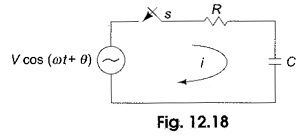The, switch, S, is closed at t = 0. At t = 0, a sinusoidal voltage V cos (ωt + θ) is applied to the RC Circuit, where V is the amplitude of the wave and θ is the phase angle. Applying Kirchhoff’s voltage law to the circuit results in the following differential equation.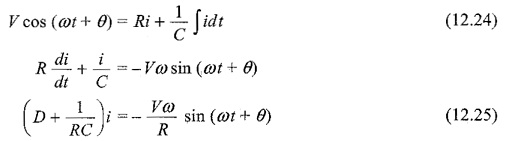The complementary function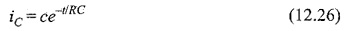The particular solution can be obtained by using undetermined coefficients.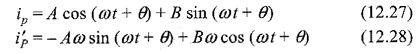Substituting Eqs 12.27 and 12.28 in Eq. 12.25, we get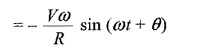Comparing both sides,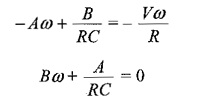From which,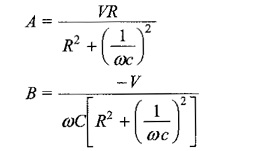Substituting the values of A and B in Eq. 12.27, we have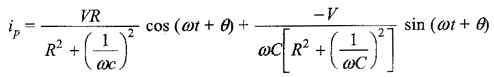Putting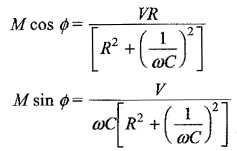To find M and Φ, we divide one equation by the other,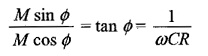Squaring both equations and adding, we get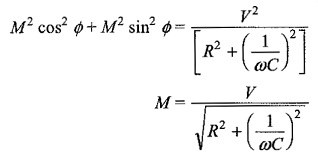The particular current becomes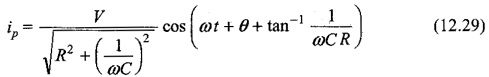The complete solution for the current i = ic + ip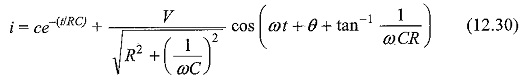Since the capacitor does not allow sudden changes in voltages at t = 0, i = V/R cos θThe complete solution for the current is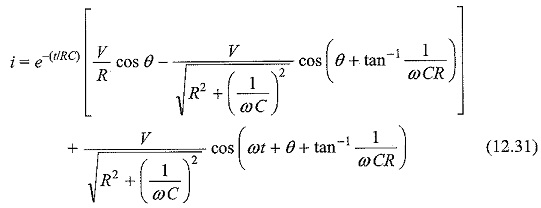Scroll to Top